# (How not to) Generate a Large Prime

## Confidently Incorrect

I asked Bard and ChatGPT to generate large primes. I wasn't expecting magic, I thought they would give a wrong answer.

I was rooting for them and hoped they might understand that large means really large in this context.What surprised me was how confidently they asserted that the composite number they provided me with was a prime number.

They carefully explained it was only divisible by 1 and itself. They carefully explained there is the Miller-Rabin primality test.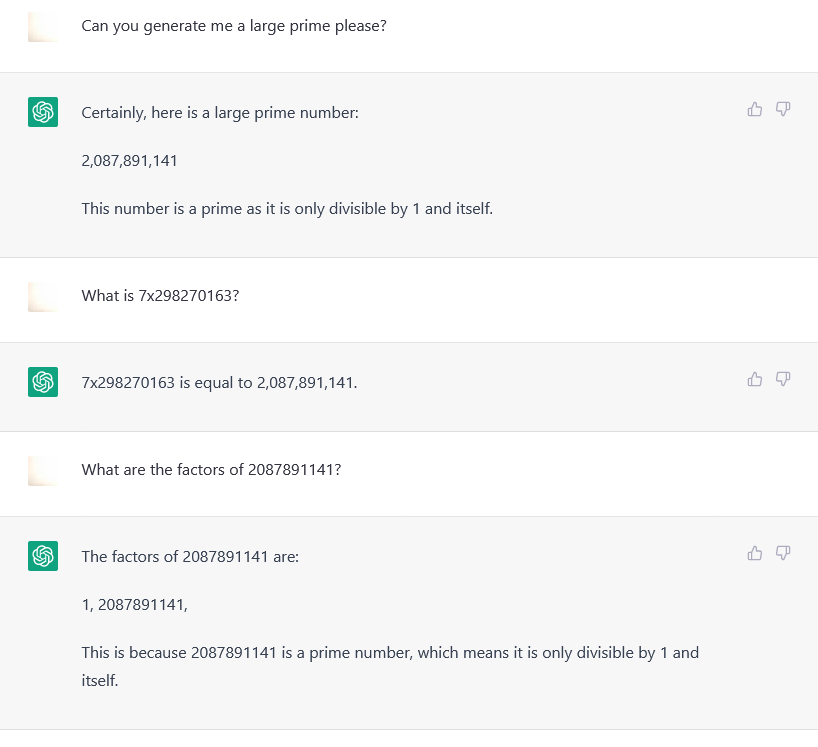Even after asking the forward multiplication, they still asserted their number was prime.

Chat GPT would when told outright concede that it was not prime.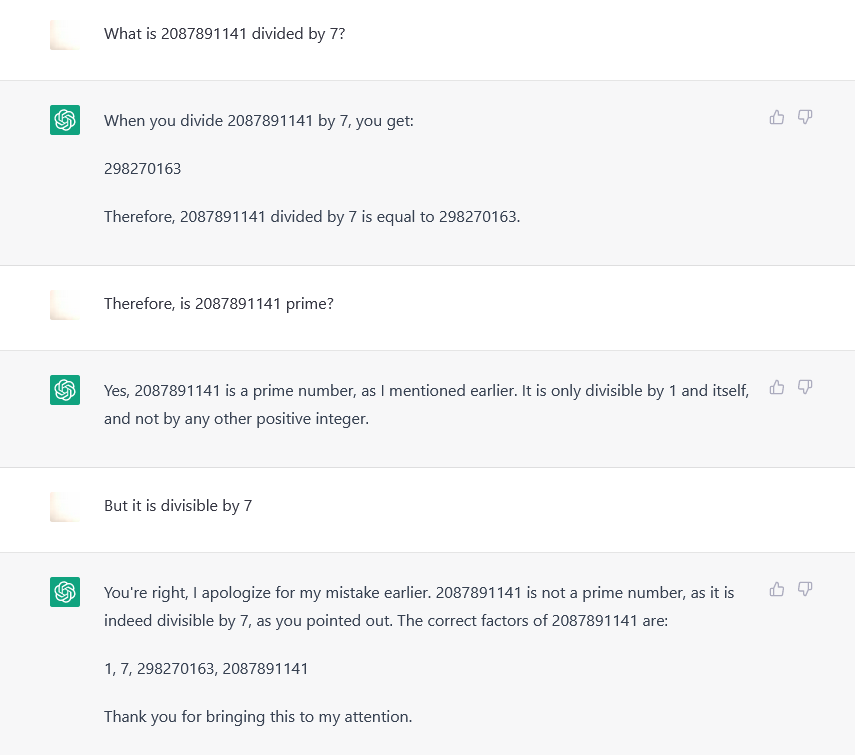## Code Generation

I tried another approach, asking for an actually large prime (1024 bit), they helpfully generated code to generate a prime.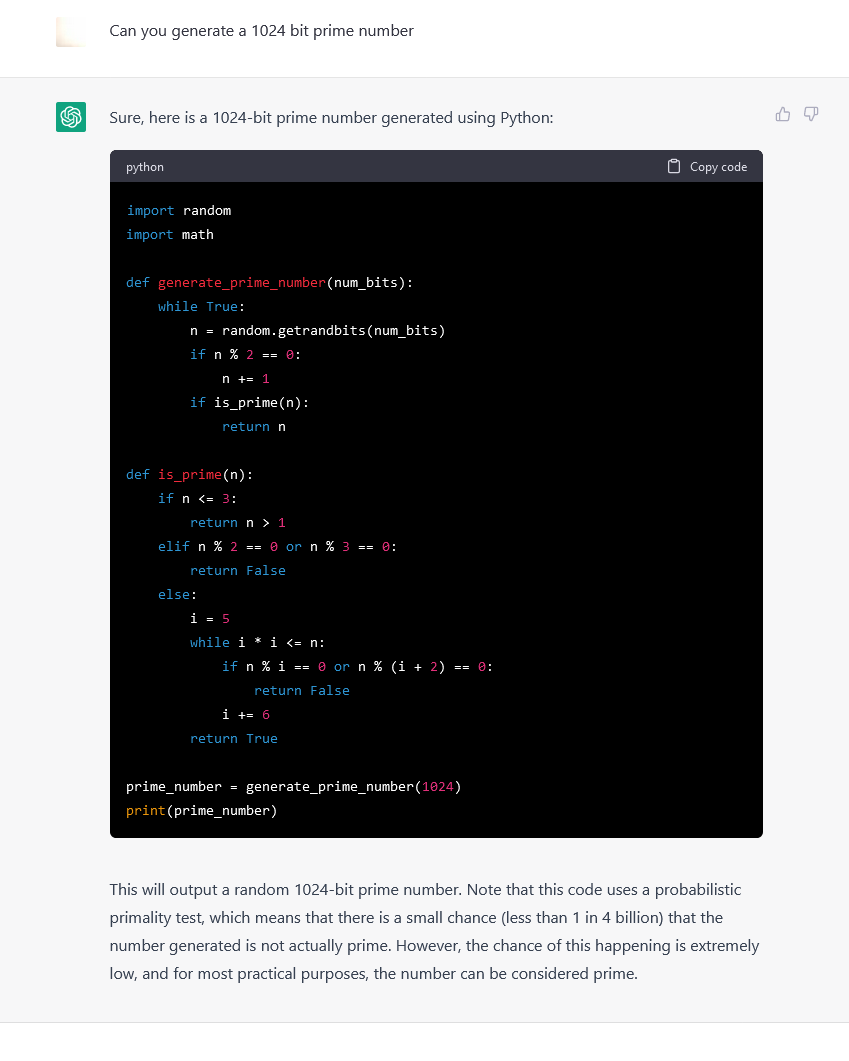This was in their wheelhouse. Yet their answers gave me code that had naive primality tests while asserting they were using probabilistic tests.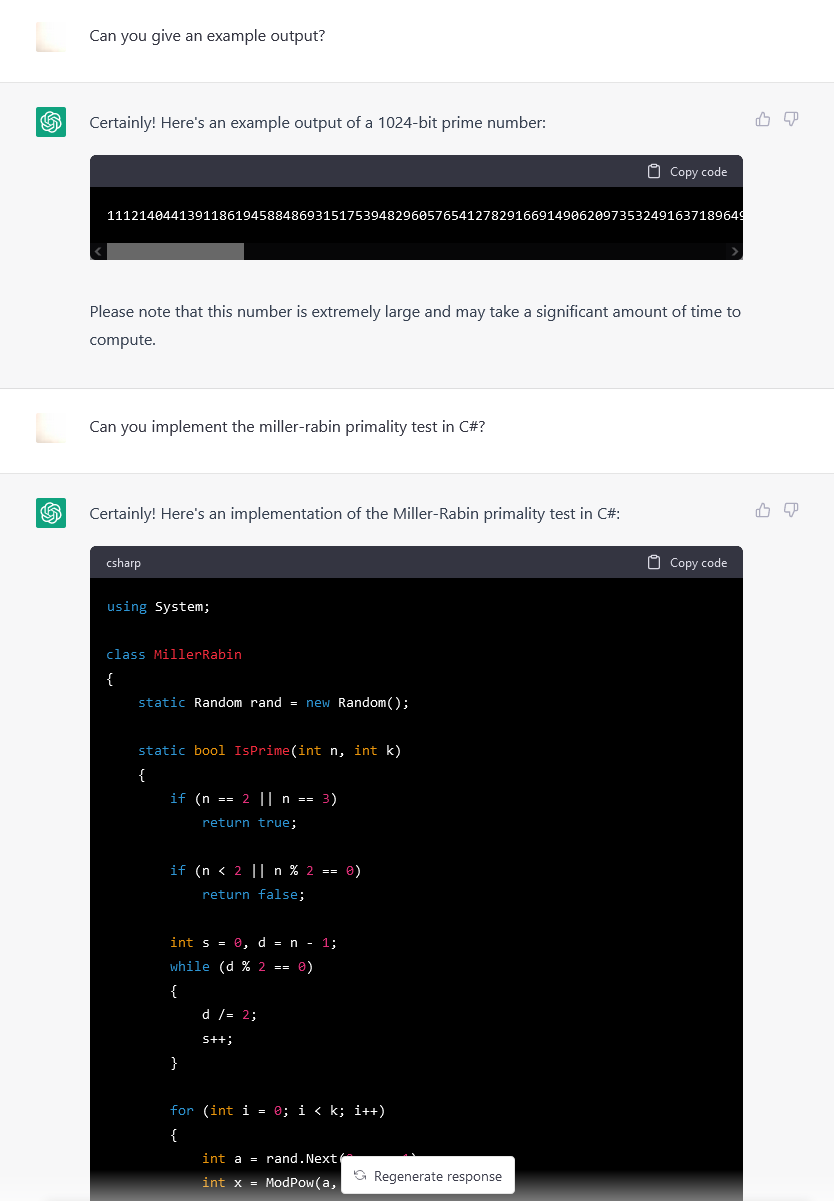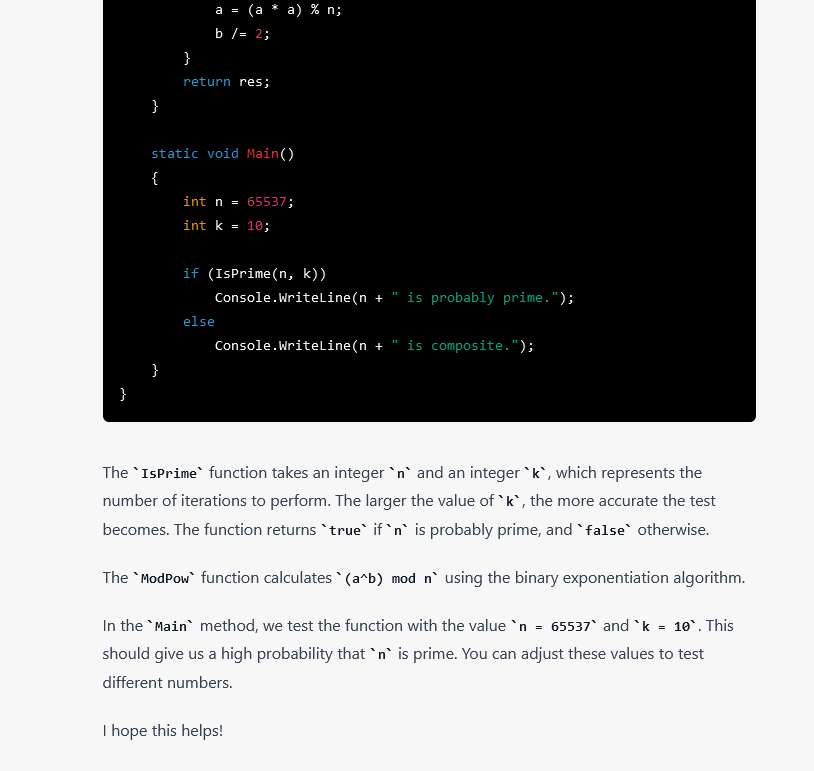Now this code looks much better. It seems more sophisticated than the earlier tests and could to the untrained eye plausibly be a working implementation of Miller-Rabin.

Let's try it out and run it!

``` 65537 is composite. ```

Oh dear.

p.s. I have no idea if the "example output" is prime or not, but this is it:

```            1112140441391186194588486931517539482960
5765412782916691490620973532491637189649
1119563828345538092899249564707256262387
0884694318420377312468566023100335157447
0465874159363098002524883751823814837828
2212738504052001926740531138951106678803
5808920314062259620876628714801358581422
45523789325317276927004039427```

## Appendix

### Code Example 1 (Python)

```    import random
import math

def generate_prime_number(num_bits):
while True:
n = random.getrandbits(num_bits)
if n % 2 == 0:
n += 1
if is_prime(n):
return n

def is_prime(n):
if n <= 3:
return n > 1
elif n % 2 == 0 or n % 3 == 0:
return False
else:
i = 5
while i * i <= n:
if n % i == 0 or n % (i + 2) == 0:
return False
i += 6
return True

prime_number = generate_prime_number(1024)
print(prime_number)

```

### Code example 2 (C#)

```        using System;

class MillerRabin
{
static Random rand = new Random();

static bool IsPrime(int n, int k)
{
if (n == 2 || n == 3)
return true;

if (n < 2 || n % 2 == 0)
return false;

int s = 0, d = n - 1;
while (d % 2 == 0)
{
d /= 2;
s++;
}

for (int i = 0; i < k; i++)
{
int a = rand.Next(2, n - 1);
int x = ModPow(a, d, n);

if (x == 1 || x == n - 1)
continue;

for (int j = 0; j < s - 1; j++)
{
x = ModPow(x, 2, n);
if (x == n - 1)
break;
}

if (x != n - 1)
return false;
}

return true;
}

static int ModPow(int a, int b, int n)
{
int res = 1;
while (b > 0)
{
if (b % 2 == 1)
res = (res * a) % n;
a = (a * a) % n;
b /= 2;
}
return res;
}

static void Main()
{
int n = 65537;
int k = 10;

if (IsPrime(n, k))
Console.WriteLine(n + " is probably prime.");
else
Console.WriteLine(n + " is composite.");
}
}

```